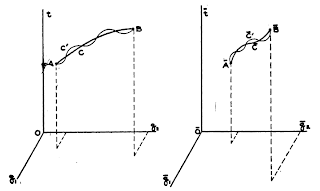# ACTION!

One more week spent on Lagrangian Dynamics...and more results!
Well, the main point about Lagrange and his ubiqutous formulae (that following level of abstraction I talked about in the last post), passes through the concept of ACTION.
Inexplicably, when learning physics at the Secondary or even at the Graduate studies, everything seems to finish when we get to the three laws of Newton.
That's the highest point one ever gets...and then we reach the concept of energy by integrating forces along paths.
But there is still one step further (or maybe more, now that the world seems to be round instead of flat, one never knows...).
The notion of action is that of the integral of the energy along time. Easy, isn't it? Its units are energy·time (Joule-second in SI).
Ok then. The problem, I guess, is that in order to be able to do something useful with it, one has to go also one step further in the mathematical notion of function: that of Functional.
And perhaps here is where our poor brains become at risk of melting...or maybe not, because a functional is just a function where the variable is...a function.
So, we are supposed to understand and memorize the linearity property of the integrals, and not the idea of functional? Come on...
Anyway, let's forgive our poor teachers of calculus and physics, who did their best I am sure, and get to work...
The discipline in charge to explain functionals is Variational Calculus, a fairly complex matter. When applied to physics, it happens to produce the Variational Principle of Physics, which is on where all Lagrangian, Jacobian, Hamiltonian and Eulerian dynamics rest.
So , in order to properly grasp the ideas of Lagrangian Dynamics, we better start with a little revision. The best one I found is from Mr. Claude Lacoursière's thesis "Ghost and machines: Regularized Variational Method for Interactive Simulation Multibodyes with dry friction" , combined with the following extremely clear explanation of the concept of action (http://wapedia.mobi/en/Action_(physics)):
1.-Work and Energy:
When a force is applied over a mass along a trajectory kinetic energy is dissipated. Integrating the formula, we can obtain its value as:
T(x')=(m·||x'||²)/2
where x' (aka velocity) is the derivative of the position, m is the mass of the particle and T(x') is the total kinetic energy.
For the cases when the force assumes values tangent to the energy function, then another form of energy appears: this is potential energy.
This special form of energy has the special property of being independent of the trajectory.And this form of energy must also be considered into our energy equation if we want to account for everything.
This leaves our equation for the energy of the system the following way:
E=T(x')+V(x)
Being T(x') the kinetic energy described above and V(x) the potential energy.
2.-Basic Variational principle:

Once we have our Energy Equation, we are able to define the Action S for that Energy.
This would be, as explained above, the integral of this equation over time.

Here, t1 and t2 are the initial and final time positions, and L(x,x') is our LAGRANGIAN, which is exactly our Energy Equation, but with another name.

The funny thing about this integral is that it solves nothing by itself. There is a lot of possible solutions for our trajectory, all of them valid in mathematical terms, but only one of them is good for us.The D'Alembert principle states that, "for a physical trajectory and infinitesimal displacements thereof at each point in time, and compatible with all imposed constraints, the work done by the virtual displacements along the trajectory vanishes when the motion is not bounded, and non-positive otherwise".
In plain English: the path followed is going to be that for which the action is minimized (or more strictly, for which the action is stationary, i.e. there is a minimum point).

To express the constraints mentioned by monsieur D'Alembert, all we need is to apply Euler-Lagrange equation:

∂L/∂xi - d/dt ( ∂L/∂xi' ) = 0

And then use the mathematical technique of the Lagrangian Multipliers (which I will not explain here), in order to simplify our Action Integral into something we can operate with, i.e. a second order differential equation.

And this is it. The basis, the cornerstone of Lagrangian Dynamics, demystified.
Once these concepts are comprehended, it is relatively easy to toddle around all the rest of this vast world...now at least we have legs!